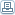# How To Calculate Your Lease Payment

 By: Gregory AshtonUnderstanding how to calculate your monthly lease payment makes it easier for you to make an informed decision. Yet, most of us shy away from the "complicated" math on our lease contract, leaving it up to the dealer to do the payment formula. Actually, it's not that difficult! Once you understand all the figures involved in calculating your monthly payments, everything else falls into place. These key figures are:MSRP (short for Manufacturer's Suggested Retail Price): This is the list price of the vehicle or the window sticker price. Money Factor: This determines the interest rate on your lease. Insist on your dealer to disclose this rate before entering into a lease. Lease Term: The number of months the dealer rents the vehicle. Residual Value: The value of the vehicle at the end of the lease. Again, you can get this figure from the dealer. Now, let us calculate a sample lease payment based on a vehicle with an MSRP (sticker price) value of \$25,000 and a money factor of 0.0034 (this is usually quoted as 3.4%). The scheduled-lease is over 3 years and the estimated residual percentage is 55%.The first step is to calculate the residual value of the car. You multiply the MSRP by the residual percentage:\$20,000 X .55 = \$11,000.The car will be worth \$13,750 at the end of the lease, so you'll be using:\$20,000 - \$11,000 = \$9,000This amount of \$9,000 will be used over a 36 month lease period giving us a monthly payment of:\$9,000 / 36 = \$250.This is the first part of the monthly payment, called the monthly depreciation charge. The second part of the monthly payment, called the money factor payment, factors the interest charge. It is calculated by adding the MSRP figure to the residual value and multiplying this by the money factor:(\$20,000 + \$11,000) * 0.0034 = \$105.4Finally, we get the approximate monthly payment by adding the two figures together:\$250 + \$105.4 = \$355.4To recapitulate, the sample formula looks like this:1- Monthly Depreciation Charge:MSRP X Depreciation Percentage = Residual Value MSRP - Residual Value = Depreciation over lease term Depreciation over lease term / lease term (number of months in the lease) = monthly depreciation charge2- Monthly factor money charge(MSRP + Residual value) X Money factor = money factor payment3- Sample Monthly Payment:depreciation charge + money factor payment = monthly paymentKeep in mind that this is a simplified calculation that does not take into account taxes, fees, rebates or any other incentives. The calculation gives you a ballpark figure or a rough idea of what your lease payments for the vehicle in question should be.